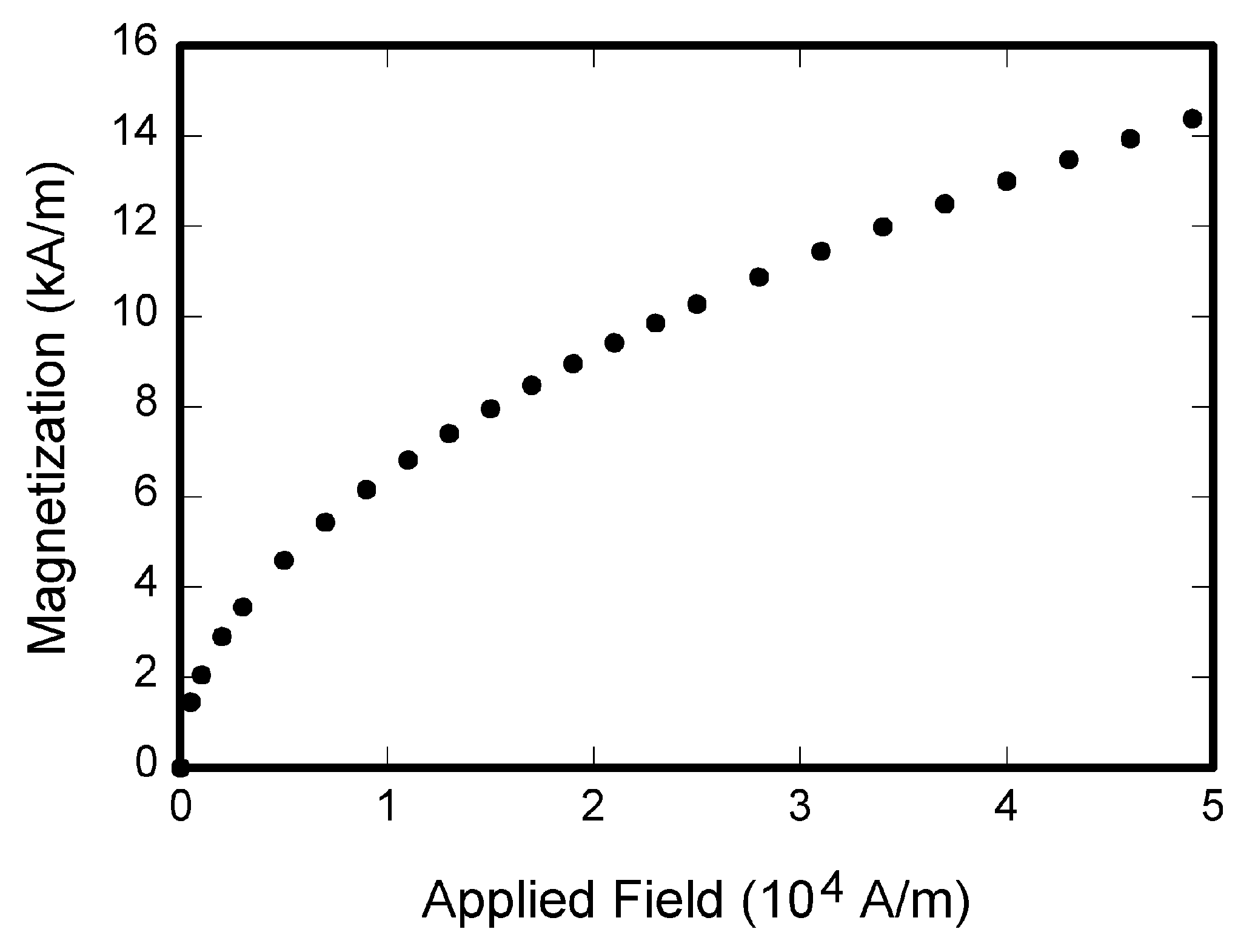#  Preparation of Papers for ieee transactions on medical imaging

Download 148.68 Kb.
 Page 3/7 Date 26.10.2016 Size 148.68 Kb.

## TABLE I Units for Magnetic Properties Symbol Quantity Conversion from Gaussian and CGS EMU to SI a  magnetic flux 1 Mx  108 Wb = 108 V·s B magnetic flux density, magnetic induction 1 G  104 T = 104 Wb/m2 H magnetic field strength 1 Oe  103/(4) A/m m magnetic moment 1 erg/G = 1 emu  103 A·m2 = 103 J/T M magnetization 1 erg/(G·cm3) = 1 emu/cm3  103 A/m 4M magnetization 1 G  103/(4) A/m  specific magnetization 1 erg/(G·g) = 1 emu/g  1 A·m2/kg j magnetic dipole moment 1 erg/G = 1 emu  4  1010 Wb·m J magnetic polarization 1 erg/(G·cm3) = 1 emu/cm3  4  104 T ,  susceptibility 1  4  mass susceptibility 1 cm3/g  4  103 m3/kg  permeability 1  4  107 H/m = 4  107 Wb/(A·m) r relative permeability   r w, W energy density 1 erg/cm3  101 J/m3 N, D demagnetizing factor 1  1/(4) Vertical lines are optional in tables. Statements that serve as captions for the entire table do not need footnote letters. aGaussian units are the same as cg emu for magnetostatics; Mx = maxwell, G = gauss, Oe = oersted; Wb = weber, V = volt, s = second, T = tesla, m = meter, A = ampere, J = joule, kg = kilogram, H = henry.Fig. 1. Magnetization as a function of applied field. Note that “Fig.” is abbreviated. There is a period after the figure number, followed by two spaces. It is good practice to explain the significance of the figure in the caption.

The database is protected by copyright ©sckool.org 2020
send message

Main page